# Scattering of Photons

In the scattering of photons, for example from an atom, an initial state photon with wave-numberand polarization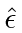is absorbed by the atom and a final state photon with wave-numberand polarization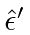is emitted. The atom may remain in the same state (elastic scattering) or it may change to another state (inelastic). Any calculation we will do will use the matrix element of the interaction Hamiltonian between initial and final states.The scattering process clearly requires terms inthat annihilate one photon and create another. The order does not matter. Theis the square of the Fourier decomposition of the radiation field so it contains terms likeand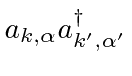which are just what we want. The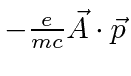term has both creation and annihilation operators in it but not products of them. It changes the number of photons by plus or minus one, not by zero as required for the scattering process. Nevertheless this part of the interaction could contribute in second order perturbation theory, by absorbing one photon in a transition from the initial atomic state to an intermediate state, then emitting another photon and making a transition to the final atomic state. While this is higher order in perturbation theory, it is the same order in the electromagnetic coupling constant, which is what really counts when expanding in powers of. Therefore, we will need to consider theterm in first order and theterm in second order perturbation theory to get an ordercalculation of the matrix element.

Start with the first order perturbation theory term. All the terms in the sum that do not annihilate the initial state photon and create the final state photon give zero. We will assume that the wavelength of the photon's is long compared to the size of the atom so that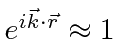.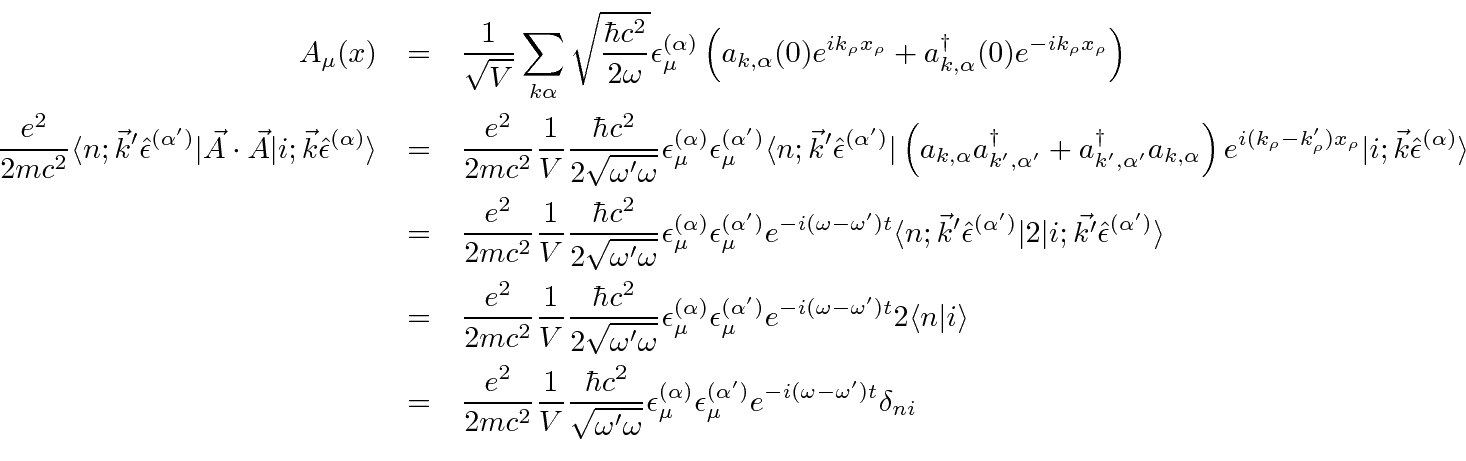This is the matrix element. The amplitude to be in the final state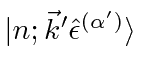is given by first order time dependent perturbation theory.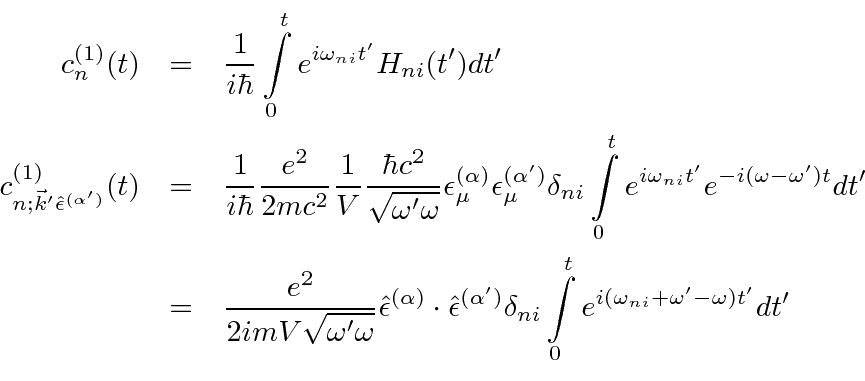Recall that the absolute square of the time integral will turn into. We will carry along the integral for now, since we are not yet ready to square it.

Now we very carefully put the interaction term into the formula for second order time dependent perturbation theory, again using. Our notation is that the intermediate state of atom and field is calledwhere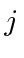represents the state of the atom and we may have zero or two photons, as indicated in the diagram.We can understand this formula as a second order transition from stateto state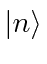through all possible intermediate states. The transition from the initial state to the intermediate state takes place at time. The transition from the intermediate state to the final state takes place at time.

The space-time diagram below shows the three terms inTime is assumed to run upward in the diagrams.Diagram (c) represents theterm in which one photon is absorbed and one emitted at the same point. Diagrams (a) and (b) represent two second order terms. In diagram (a) the initial state photon is absorbed at time, leaving the atom in an intermediate state which may or may not be the same as the initial (or final) atomic state. This intermediate state has no photons in the field. In diagram (b), the atom emits the final state photon at time, leaving the atom in some intermediate state. The intermediate state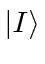includes two photons in the field for this diagram. At timethe atom absorbs the initial state photon.

Looking again at the formula for the second order scattering amplitude, note that we integrate over the timesandand that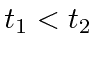. For diagram (a), the annihilation operator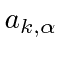is active at timeand the creation operator is active at time. For diagram (b) its just the opposite. The second order formula above contains four terms as written. Theandterms are the ones described by the diagram. Theandterms will clearly give zero. Note that we are just picking the terms that will survive the calculation, not changing any formulas.

Now, reduce to the two nonzero terms. The operators just give a factor ofand make the photon states work out. Ifis the intermediate atomic state, the second order term reduces to.Theterms coming from the integration overcan be dropped. We can anticipate that the integral overwill eventually give us a delta function of energy conservation, going to infinity when energy is conserved and going to zero when it is not. Thoseterms can never go to infinity and can therefore be neglected. When the energy conservation is satisfied, those terms are negligible and when it is not, the whole thing goes to zero.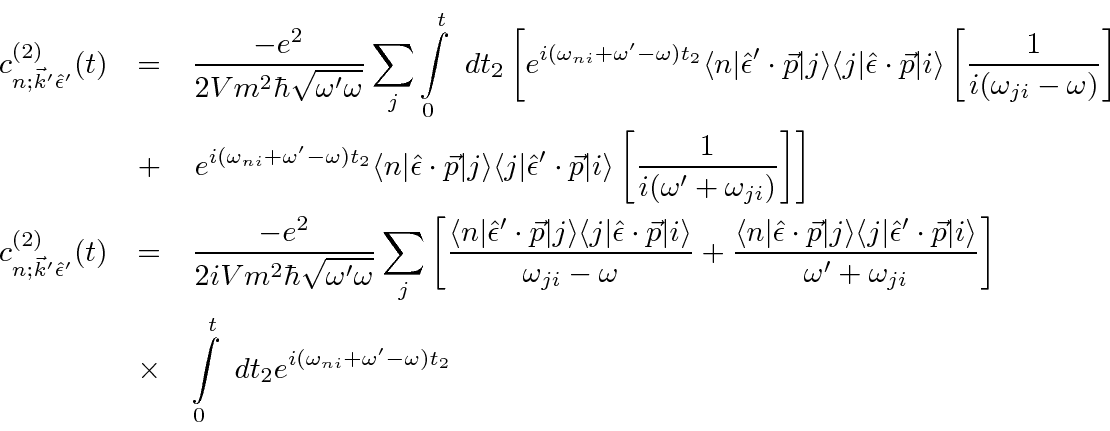We have calculated all the amplitudes. The first order and second order amplitudes should be combined, then squared.Note that the delta function has enforced energy conservation requiring that, but we have leftin the formula for convenience.

The final step to a differential cross section is to divide the transition rate by the incident flux of particles. This is a surprisingly easy step because we are using plane waves of photons. The initial state is one particle in the volumemoving with a velocity of, so the flux is simply.The classical radius of the electron is defined to be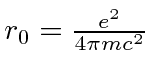in our units. We will factor the square of this out but leave the answer in terms of fundamental constants.This is called the Kramers-Heisenberg Formula. Even now, the three (space-time) Feynman diagrams are visible as separate terms in the formula.(They show up like.) Note that, for the very short time that the system is in an intermediate state, energy conservation is not strictly enforced. The energy denominators in the formula suppress larger energy non-conservation. The formula can be applied to several physical situations as discussed below.

Also note that the formula yields an infinite result if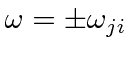. This is not a physical result. In fact the cross section will be large but not infinite when energy is conserved in the intermediate state. This condition is often refereed to as the intermediate state being on the mass shell'' because of the relation between energy and mass in four dimensions.

Subsections
Jim Branson 2013-04-22